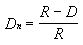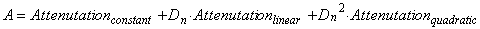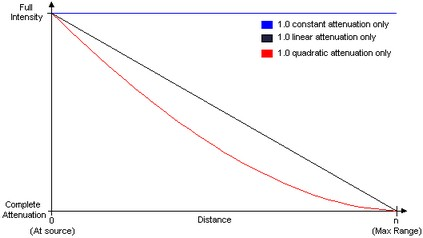﻿ 20-sim webhelp > Toolboxes > Animation Toolbox > 3D Animation > Properties > Attenuation

# Attenuation

The Animation Engine in 20-sim is based on the Microsoft® Direct3D. Direct3D uses the following formula to normalize the distance from a light source to the object surface into a value from 0.0 to 1.0, inclusive:In the preceding formula, Dn is the normalized distance, R is the light's range, and D is the distance, in world space, from the light source to the object surface. A normalized distance is 1.0 at the light's source, and 0.0 at the light's range.

With the normalized distance in hand, Direct3D then applies the following formula to calculate light attenuation over distance for point lights and spotlights:In this attenuation formula, A is the calculated total attenuation and Dn is the normalized distance from the light source to the object. The constant, linear and quadratic attenuation factors act as coefficients in the formula. You can produce a wide variety of attenuation curves by making simple adjustments to them. Most applications will set the linear attenuation factor to 1.0 and set the remaining factors to 0.0 to produce a light that steadily falls off over distance. Similarly, you could apply a constant attenuation factor of 1.0 by itself to make a light that doesn't attenuate (but will still be limited by range). The following illustration shows the three most common attenuation curves.